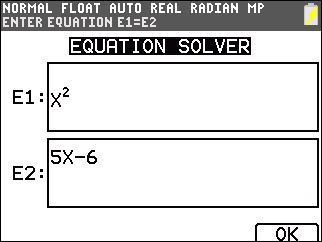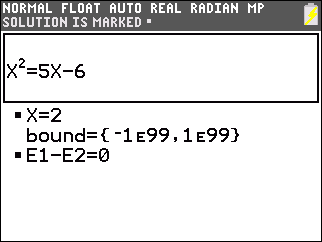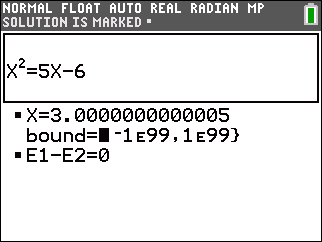# Knowledge Base

## Solution 34534: Solving a Quadratic Equation Using Numeric Solver on the TI-84 Plus CE and TI-84 Plus C Silver Edition.

### How can I solve a quadratic equation using Numeric Solver on the TI-84 Plus CE and TI-84 Plus C Silver Edition?

The numeric "Solver" feature is limited to solving for only one solution at a time. Since there are generally two solutions for a quadratic equation, two different guesses must be entered into the solver to find both solutions. After finding the first solution, enter a new initial guess or new bounds to look for the second solution.

Follow the steps below to solve the example equation: x2=5x-6.

1) Press [MATH] [ALPHA] [B].
2) Once you will the two boxes, E1 and E2, press [x] [x2] next to E1.
3) Arrow down to E2 and press  [x] [-]  then press [GRAPH] to select OK.4) Notice that the equation is now written correctly.
5) Input the first guess next to x=.
6) Press [ALPHA] [ENTER] to select "SOLVE" and the first answer will appear.Input the second guess next to x=

7) Press [ALPHA] [ENTER] to select "SOLVE" and the second answer will appear.Please see the TI-84 Plus CE and TI-84 Plus C Silver Edition guidebooks for additional information.